# Number Chart

In maths, numbers play an important role as it is the basic requirement to deal with any concept. Numbers are introduced to students from elementary level to make them understand the patterns. At this level, we use different number charts for kids to help them easily memorise the counting of numbers. In this article, you will learn the definition of numbers chart, different patterns in numbers chart along with practice questions.

What is a Number Chart?

A numbers chart is defined as a table that lists the numbers in numerical order. This order will be like 1-10 in the first line, 11-20 in the second line, 21-30 in the third line and so on. There can be different kinds of specific numbers charts, such as hundred’s charts that contain the numerals from 1–100. These charts can help students identify numerals and the numerical order and establish patterns within the numbers. For example, even numbers, odd numbers, counting by fives, tens, etc. Some of the number charts may only have the written numerals or contain the integers with a picture representation of the number of objects with each digit.

## Number Chart 1 to 100

The below figure represents the number chart from 1 to 100. It is also called a hundred’s chart.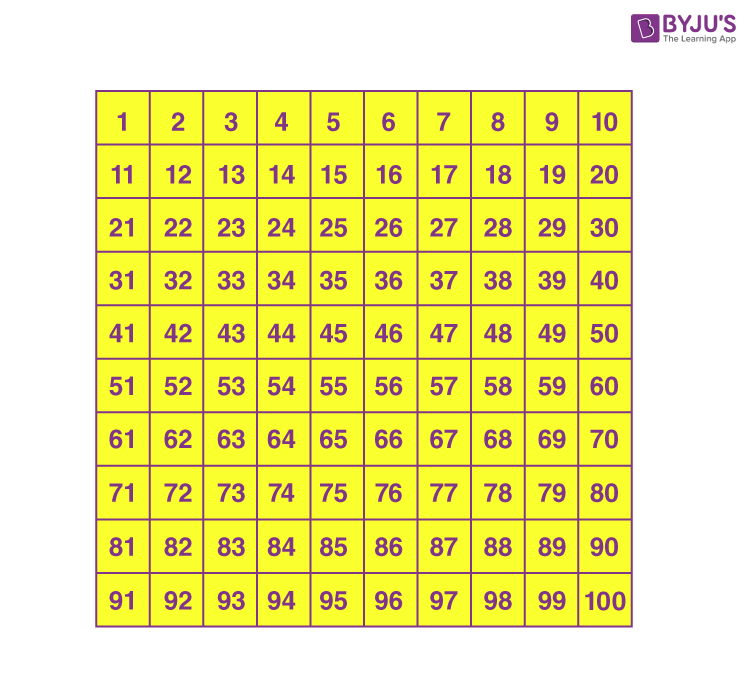### Number Chart in Words

It is possible to write the numbers in words so that it is easy to understand how to read the numericals. The below chart shows the numbers from 1 to 20 along with the words, i.e. the value of numerical in words.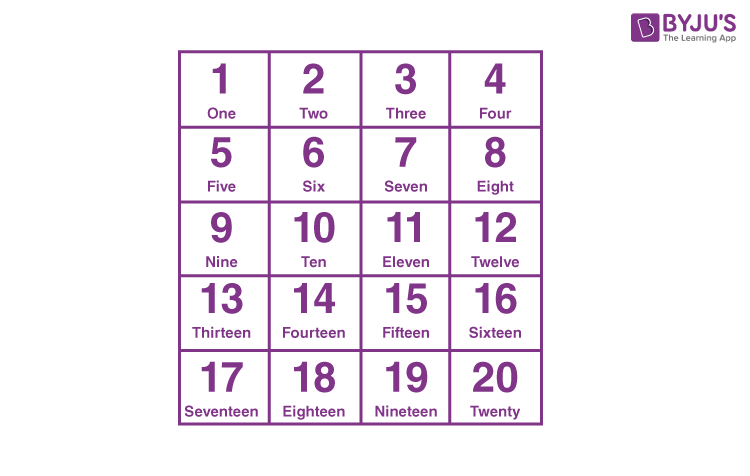## Number Chart Patterns

We can observe different patterns in number charts such as even numbers, odd numbers, prime numbers and so on. The below figure shows the even numbers from 1 to 100, where the empty spaces should be filled with odd numbers.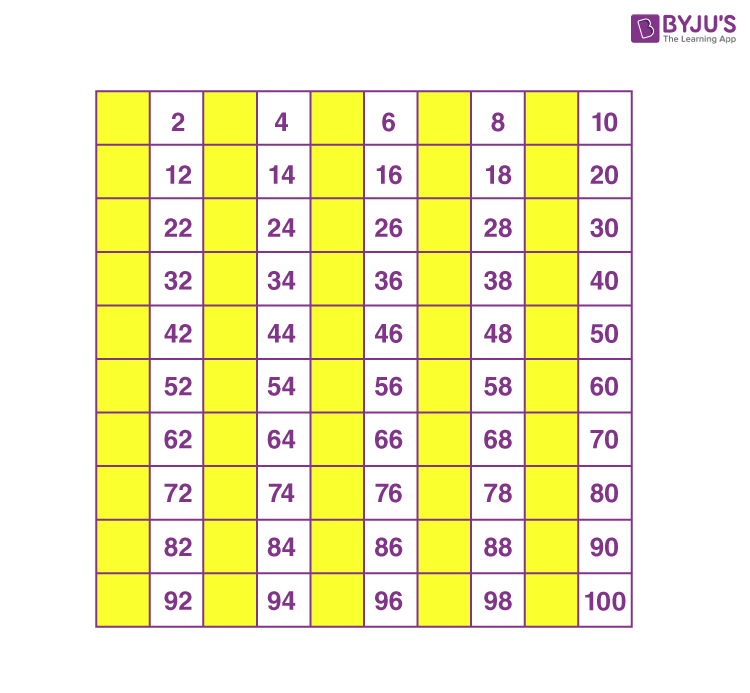Now, let’s have a look at the chart of odd numbers, where we have to fill the empty cells with even numbers.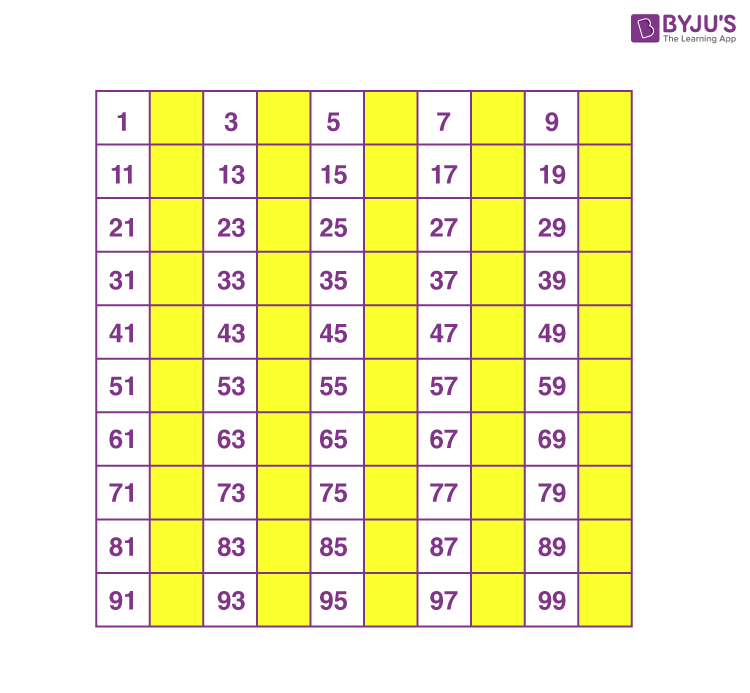### Number Chart 500 to 1000

In this section, you can get the number chart from 501 to 1000. This chart is given in the downloadable pdf format.

Apart from the above given numbers charts, we can also create charts based on skip counting. Below are a few practice questions on completing number charts based on different patterns.

1. Complete the chart given below.

Starting at 1, skip-count by 3, and fill in the missing numbers.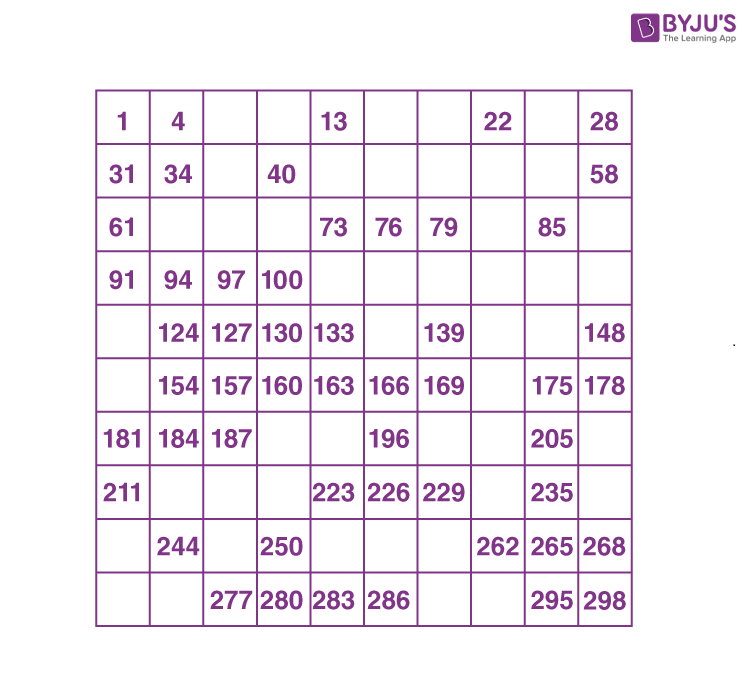2. Identify the missing numbers in the below chart.

Starting at 5, skip-count 5, and fill in the missing numbers.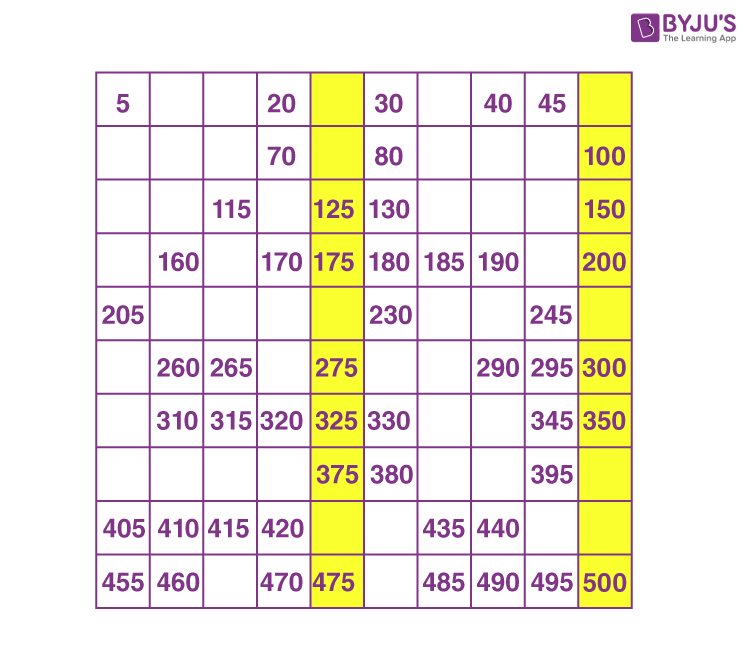3. Identify the pattern and write the numbers in the missing places.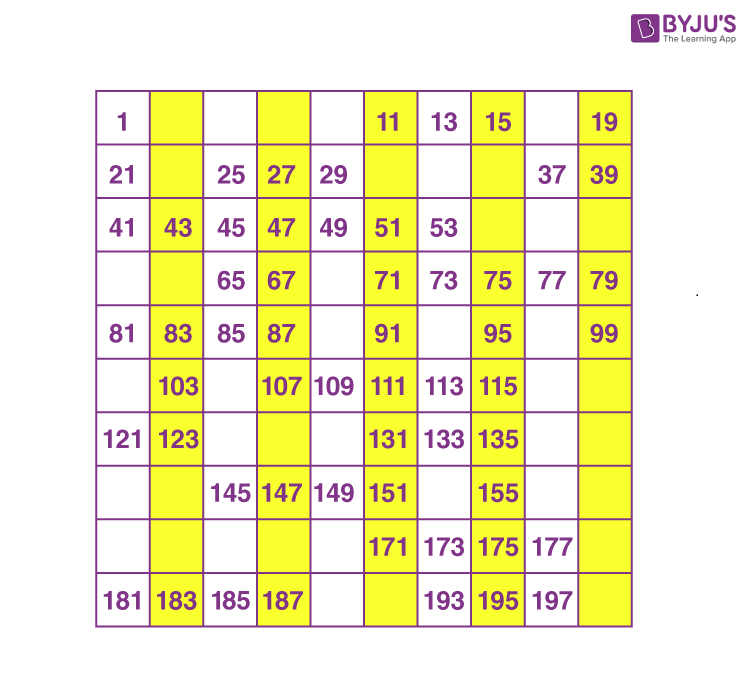#### Watch The Below Video To Know about Number PatternsVisit www.byjus.com for more information on charts and patterns on numbers. Also, download BYJU’S – The Learning App for interactive videos on number patterns.